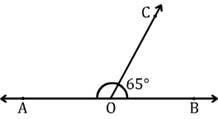# In the figure below, AB is a straight line and ∠BOC = 65 o . The measure of ∠AOC is ____.A) 145 o B) 115 o C) 125 o D) 165 o

In the figure AB is a straight line & angle BOC & angle AOC are supplementary angles.

When the sum of two angles equal to 180 Deg then the angles are called supplementary angles.

So the angle AOC = 180 - 65 = 115 Deg.

Ans.: B = 115 Deg.

• 1
What are you looking for?